Chapter 4 Part 2 - Income Determination and Multiplier

Economics Class 12
Macroeconomics

## How to Determine Equilibrium Level of income

According to Keynes

Equilibrium Level of Income is determined at a point where

Aggregate Demand is equal to Aggregate Supply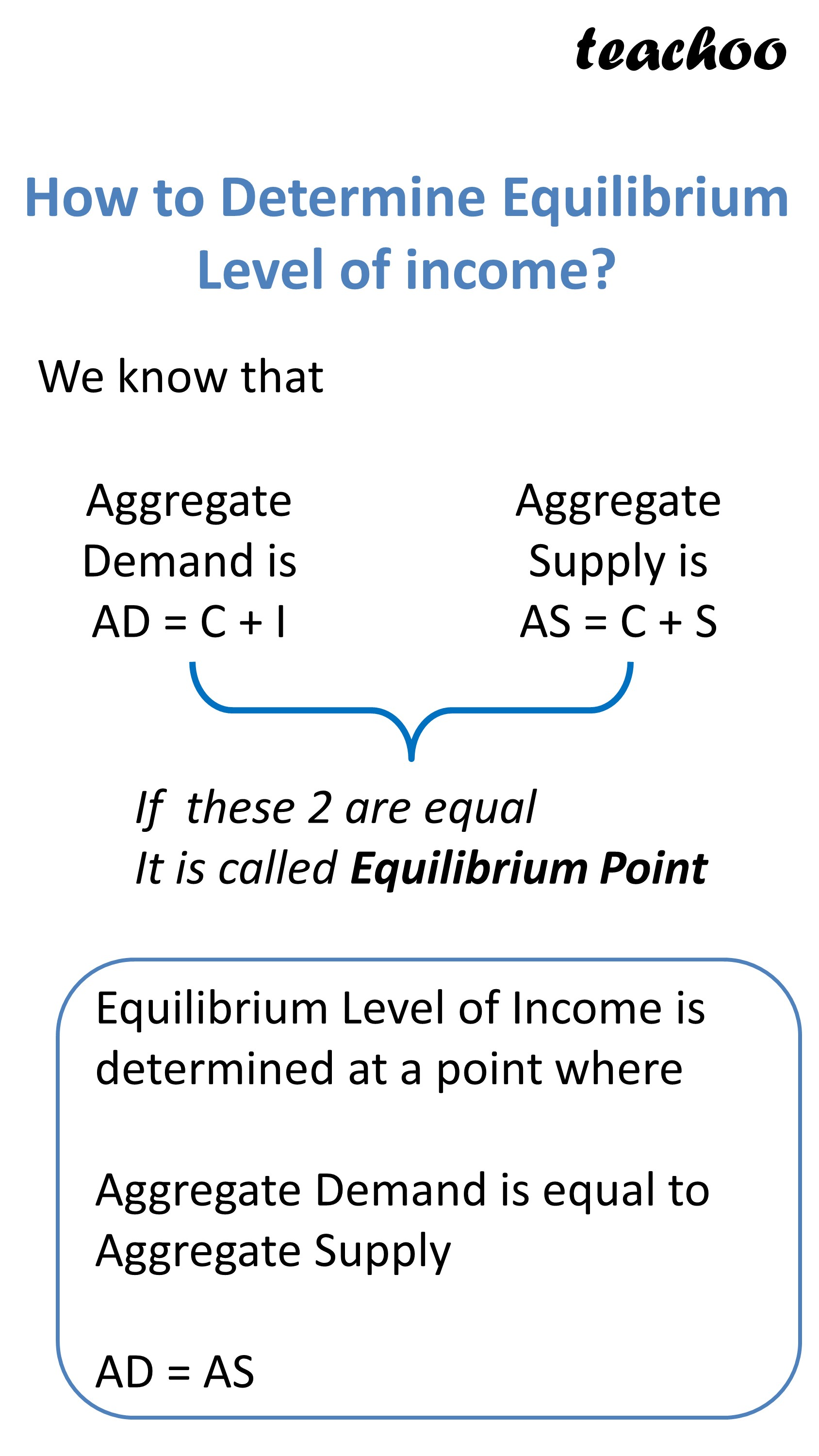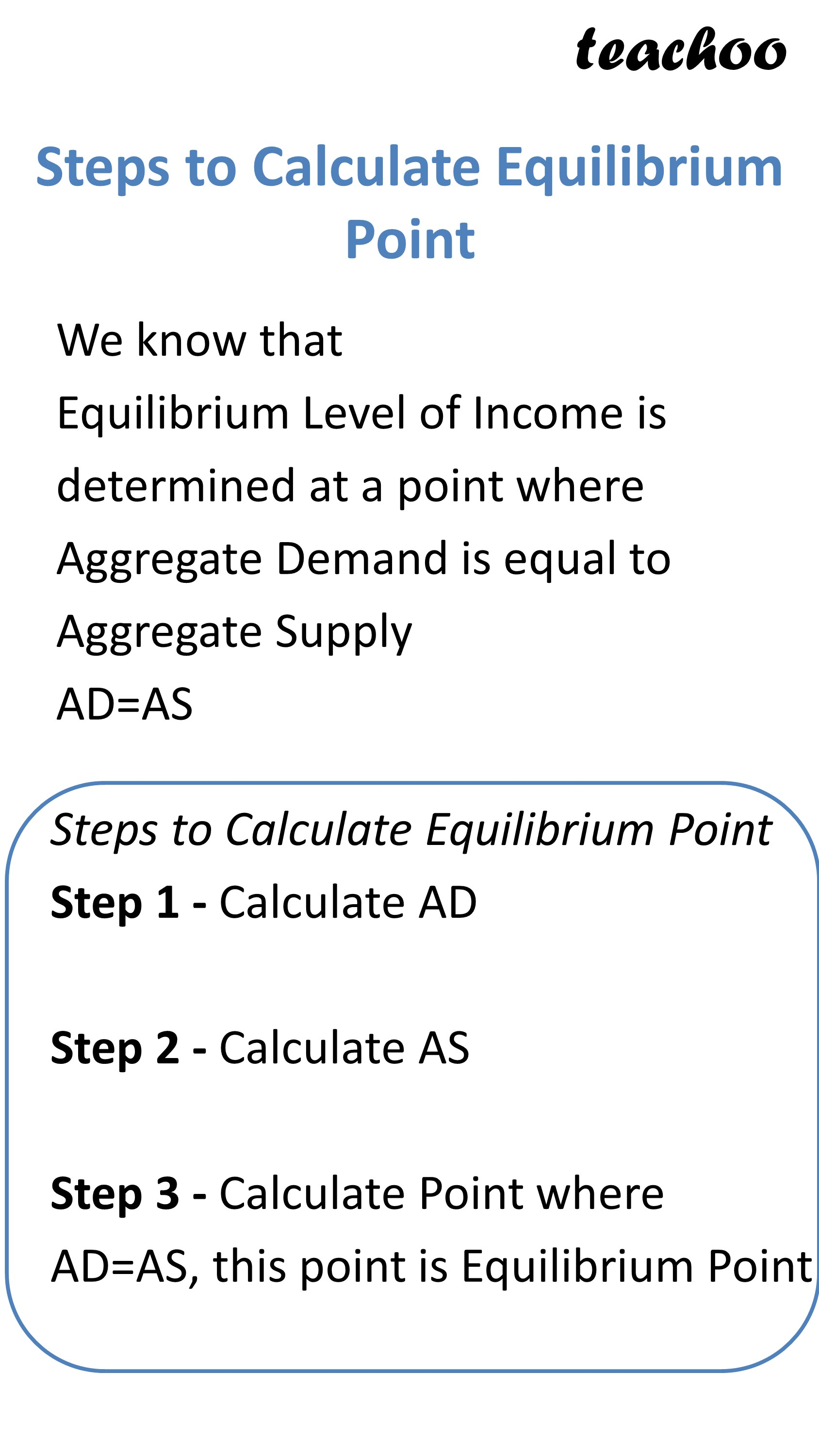Explanation

## Aggregate Demand is

It means total demand of final goods and services of all sectors of an economy at a given level of income

Aggregate Demand = Consumption Exp + Investment Expenditure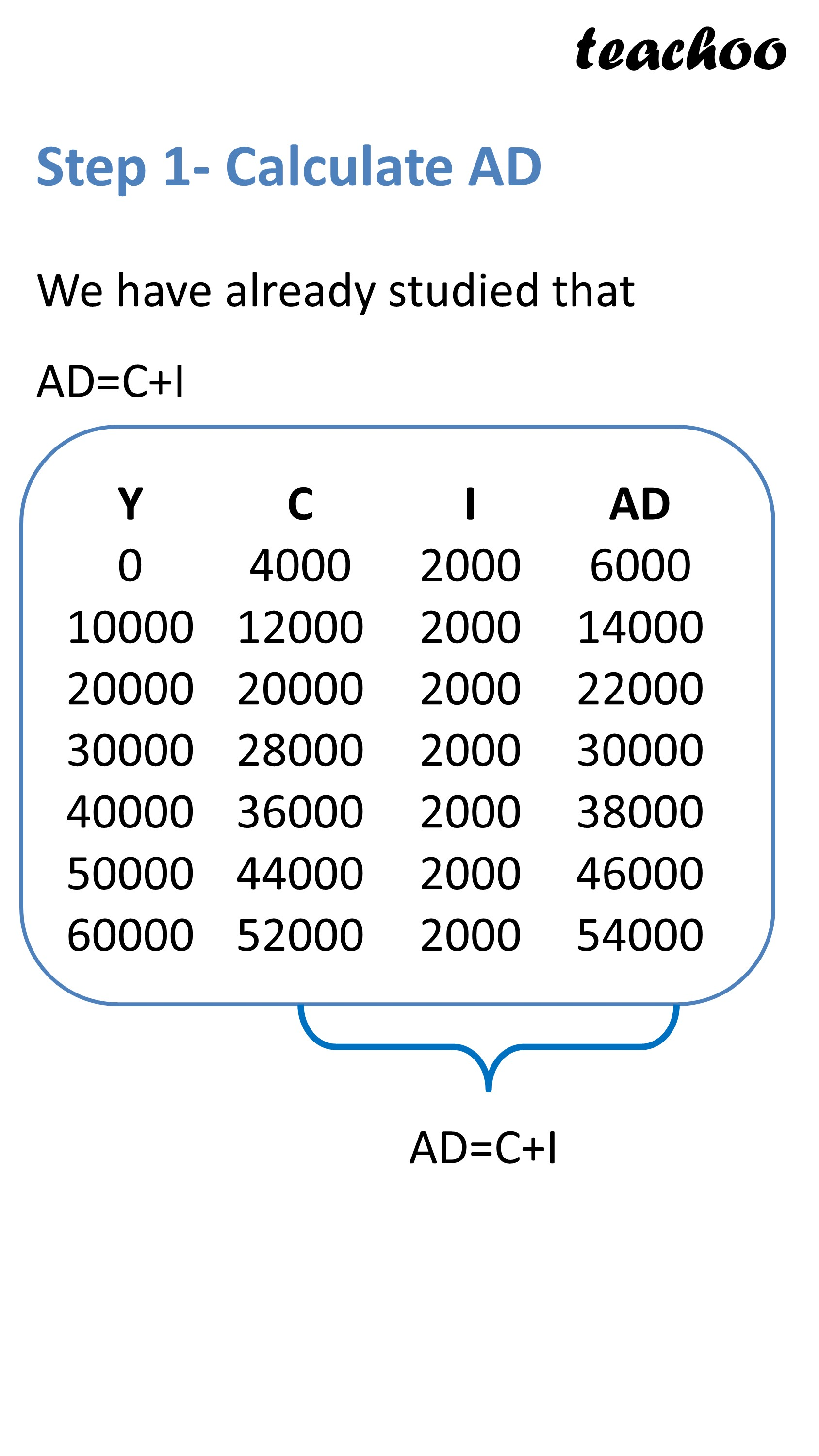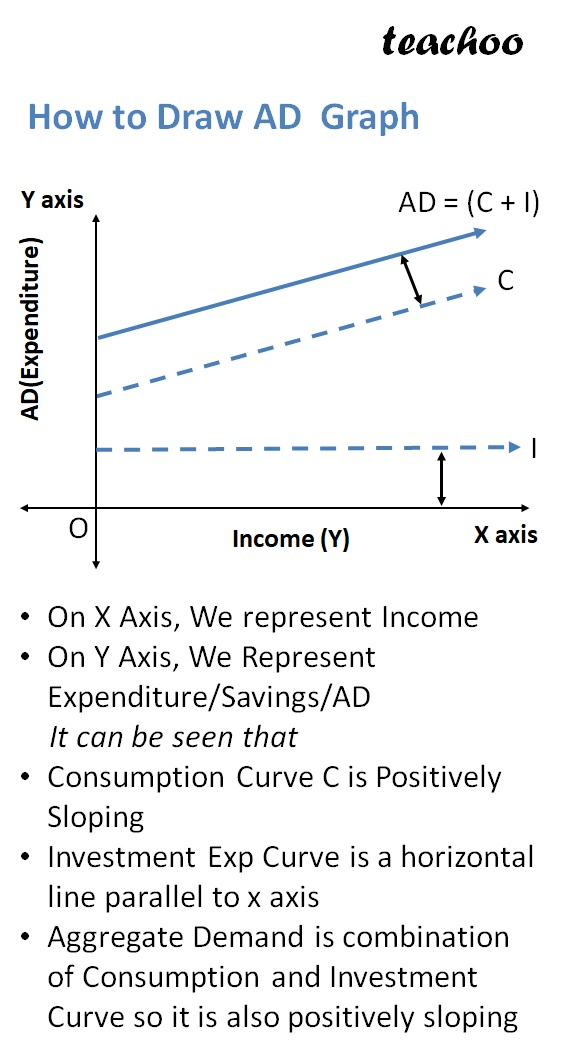## Aggregate Supply

Aggregate Supply means

total money value of goods and services

which all producers in economy

are willing to supply

Note

Aggregate Supply = National Income

AS = Y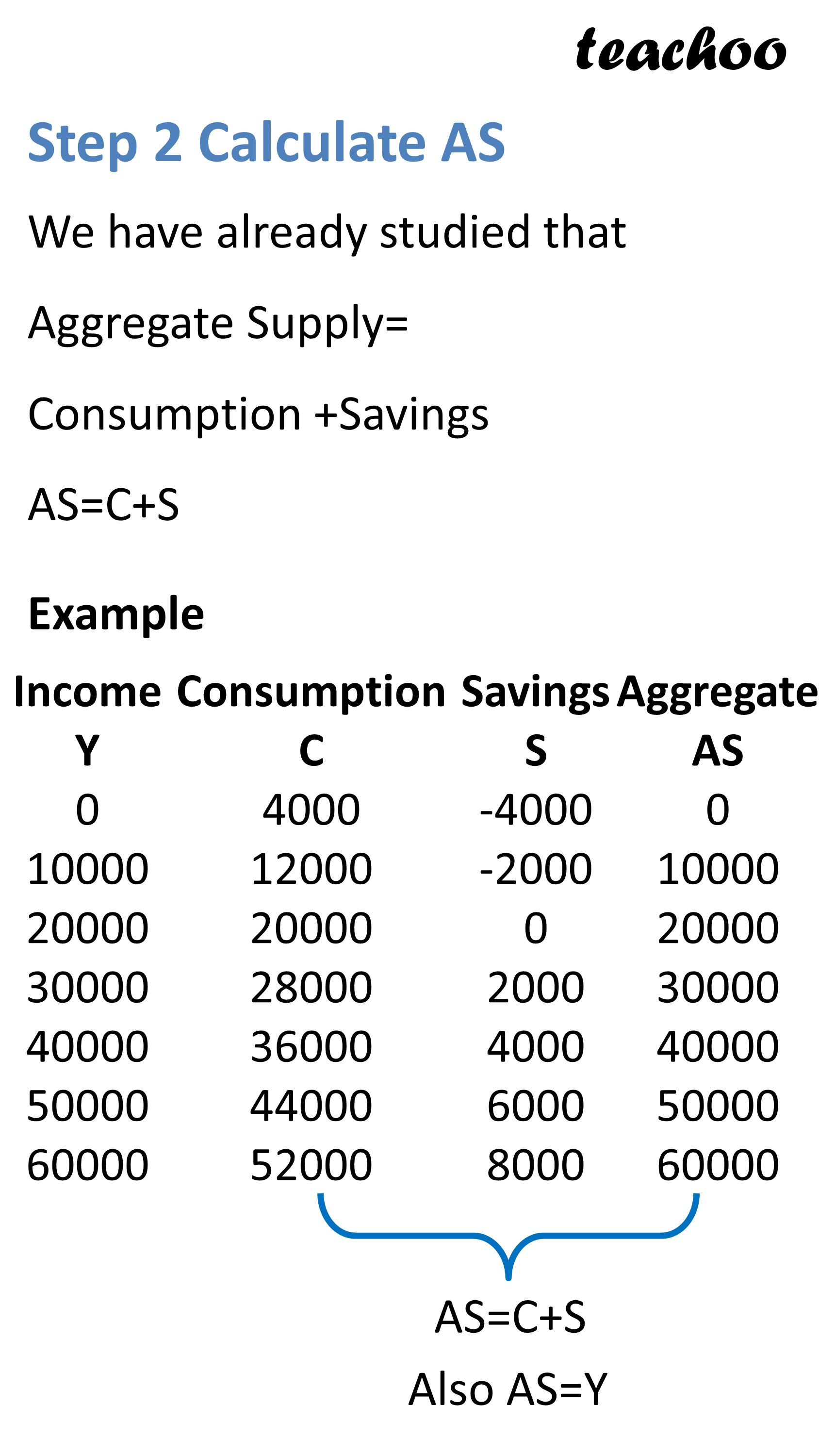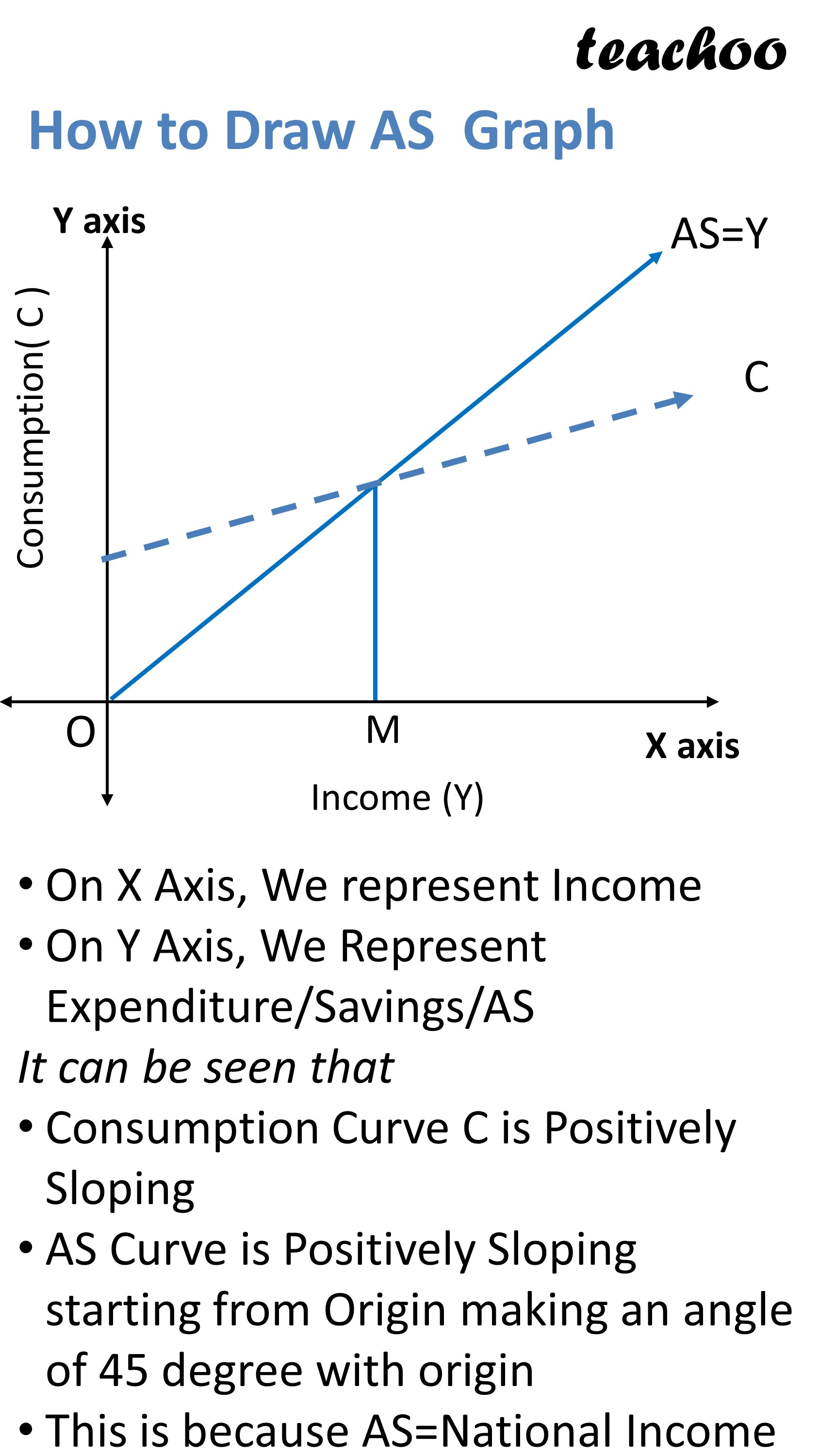## Equilibrium Point is Determined at point where

Aggregate Demand = Aggregate Supply

Hence, Value of these 2 should be equal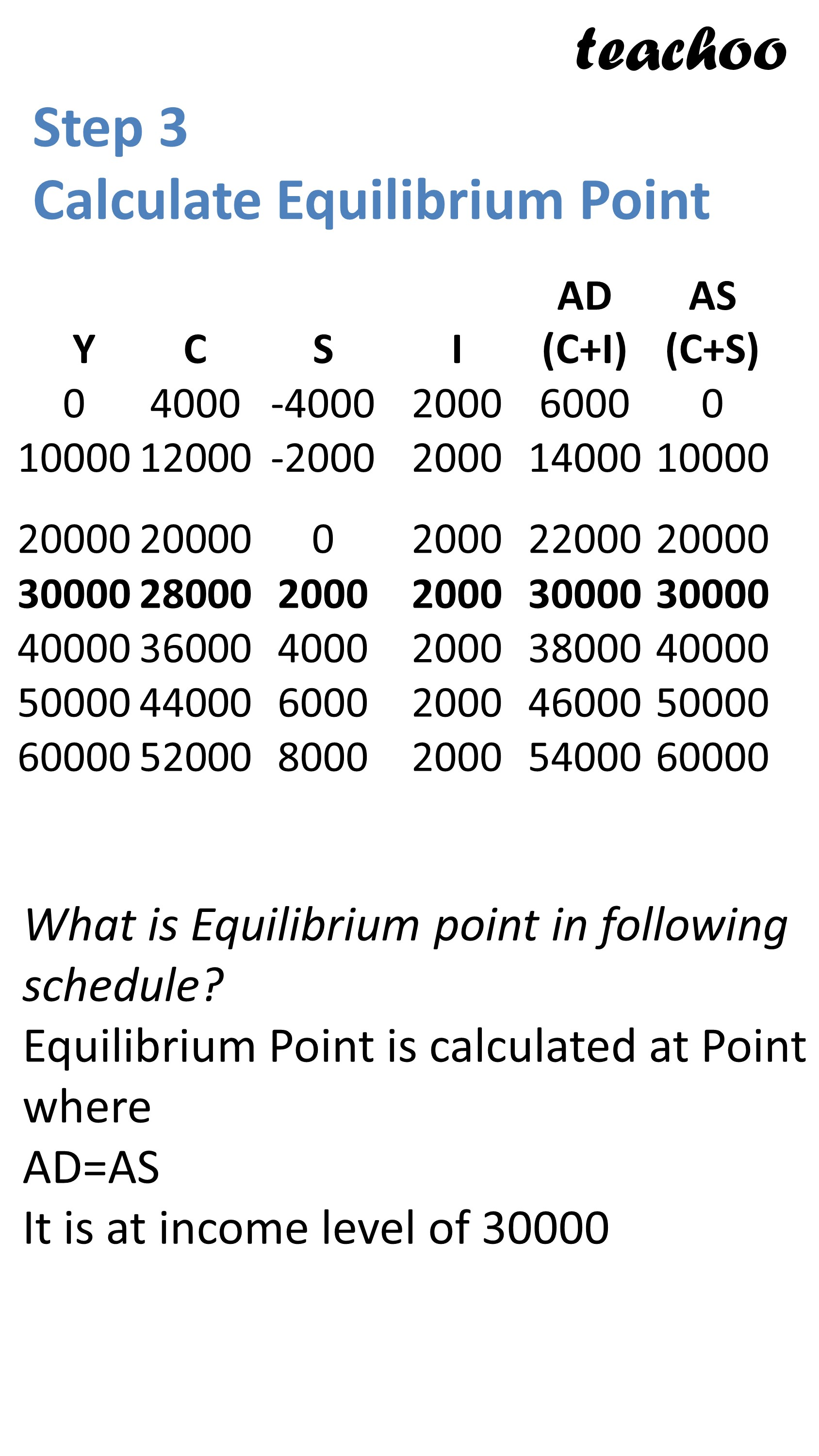## How to Make Graph of AD and AS?

On X Axis, we show Income

On Y Axis, we show AD and AS

It can be Seen that

AD is Positively Sloping Starting from Point M

AS is also Positively Sloping but starts from Point 0.

It makes an angle of 45 Degree with Origin

Point where AD and AS Curve intersect is Equilibrium Point

At this Point, we draw a line EY connecting x axis at Point Y

This Y is Equilibrium Level of income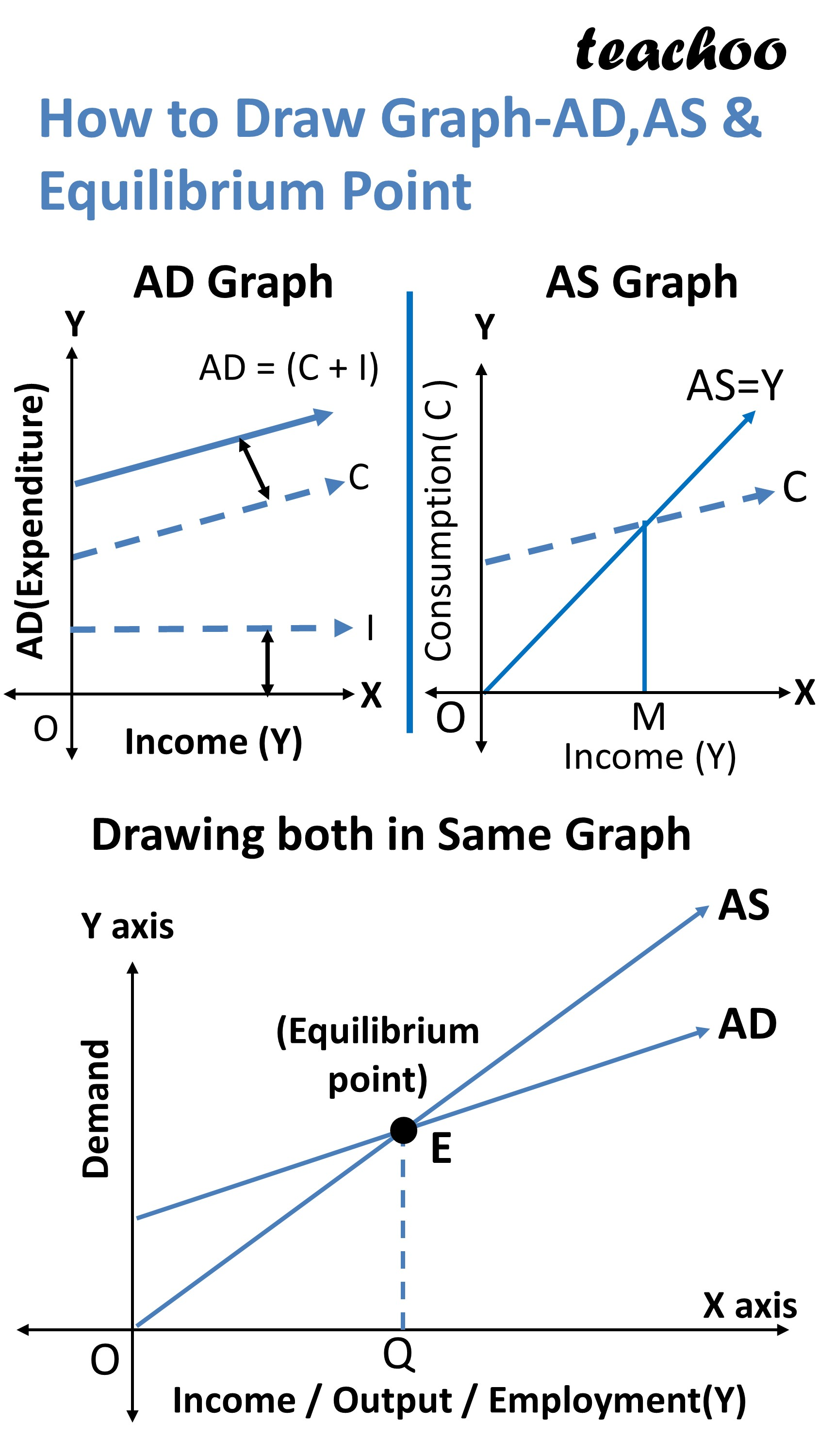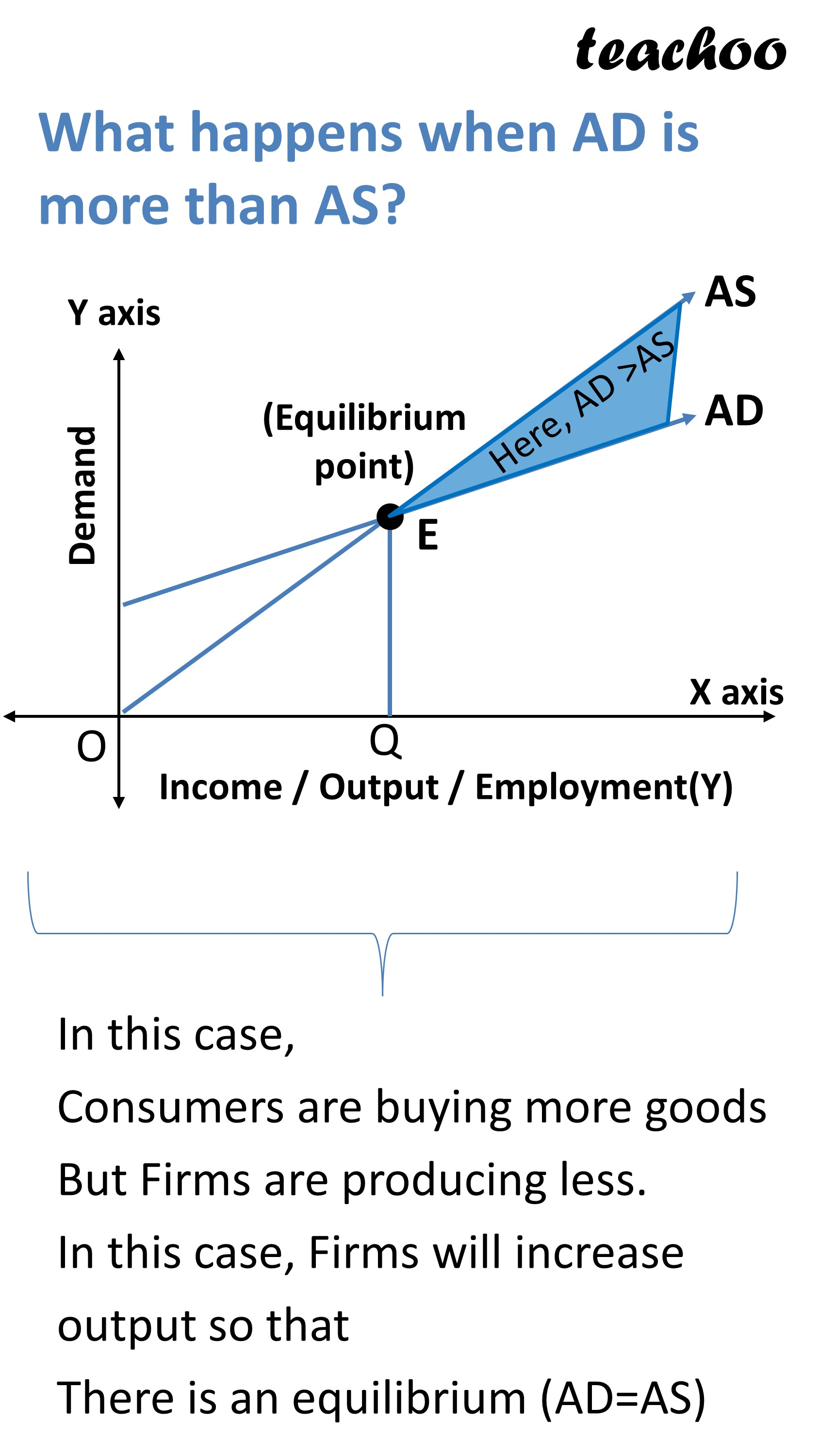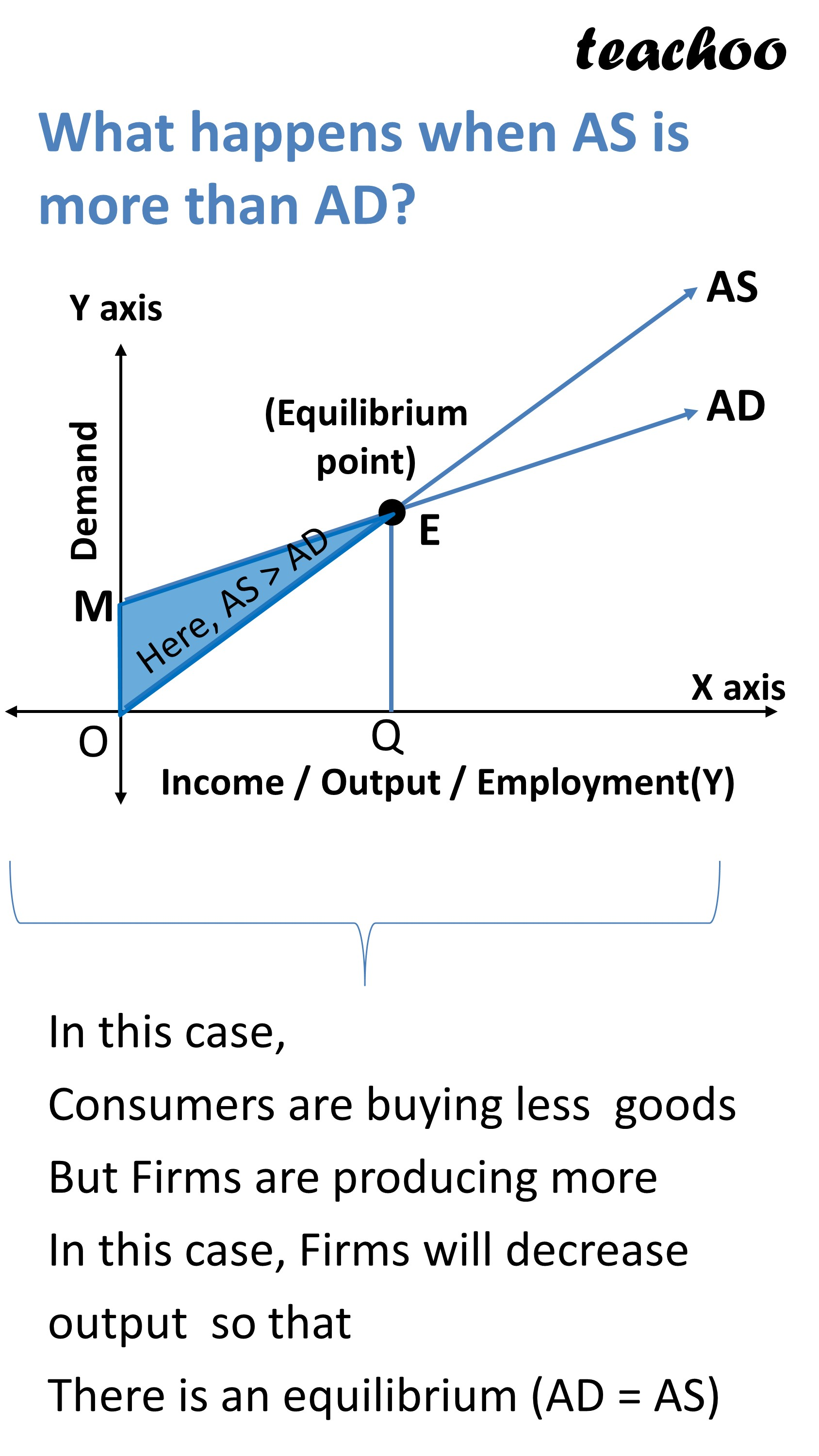### Example 22

Calculate Savings, AD, AS and determine equilibrium level if investment is fixed at Rs100 crores.

 Income 0 100 200 300 400 500 Consumption 50 100 150 200 250 300

### Example 23

Suppose investment is Rs 40 crores.

The consumption expenditure, at different levels of income, is given below.

 Income 200 300 400 500 600 700 Consumption 220 300 380 460 540 620
2. At what level of income will the economy be in equilibrium?
3. State the level of income where total saving is negative
4. Are saving and investment equal at equilibrium level of income?
5. Calculate MPC and MPS, when the income increases from Rs400 crores to Rs500 crores
6. What will be the AD at the income level of Rs 300 crores.

### NCERT Questions

No questions in this part

### Other Books

#### Question 1

How is equilibrium level of income attained through AD and AS approach?

Learn in your speed, with individual attention - Teachoo Maths 1-on-1 Class

### Transcript